Logiciel libre de géométrie, d'analyse et de simulation multiplateforme par Yves Biton

# How to get random integers all different and sort them using matrices

modification samedi 4 février 2023.

Toutes les versions de cet article : [English] [Español] [français]

For instance we wish to get five random integers in the range 1 to 10, all different, and sort them by increasing order.

Expand the calculations toolbar and, on it’s right, click on icon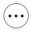. The choose item Matrix with random interger values.

Fill in the dialog box as shown here :

So our matrix will be a one row matrix with ten columns containing integers in the range 1-10 and all different.

To visualize this matrix value you can use icon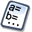(Numerical object modification) or tool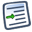(figure protocol).

If you want a display of this matrix on the figure, expand the displays toolbar and use icon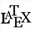(free LaTeX display).

Click on the figure on the sopt of your choice, then, in the popping up dialog box, click on button Value insertion. In the new dialog box popping up, select the matrix Mat and validate.

The LaTeX code is now : \ValMat

If you want this display to lay inside a couple of parentheses, select all the LaTeX code like here :

Then click on the icon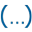(LaTeX code for parentheses).

The LaTeX code is now : \left( \ValMat \right)

Validate the dialog box.

Now let us create a matrix of one row and 5 columns containing the first five cells of matrix Mat.

Expand the calculations toolbar and click on icon. Choose item Matrix by formule.

Fill in the dialog box as shown here and validate :

This matrix is defined by a formula for each cell with row index i and column index j.
The cells will be the same as the celles of matrix Mat but this matrix will have only 5 columns.

Like before, create a LaTeX display displaying the value of this matrix under the first LaTeX display.

Here is the LaTeX code to be used : \left( \Val{MatExt} \right)

Now let us create a last matrix of 1 row and 5 columns containing the values of matrix MatExt but with theses values sorted in increasing order.

Expand the calculations toolbar and click on icon. then choose item Matricial calculation.

Fill in the dialog box as shown here :

Here is the formula to be used : sortbyrow(MatExt,1)

Function sortbyrow(mat, indrow) returns a matrix of the same dimensions as matrix mat, but the columns of matrix mat are sorted in increasing order accordingly to the values of the cells in the line number indrow (indrow may vary form 1 to the number of rows of matrix mat) .

Here the return matrix is just the same as matrix MatExt but with the cells in increasing order.

Now you can create a last LaTeX display displaying the vlue of this last Matrix.

Here is the LaTex code to be used : \left( \Val{MatSort} \right)

To be noted :

• If we had satrted with a one column matrix, we woud have used the formula sortbycol(MatExt,1)

Here is the figure you get (you can modify it) :